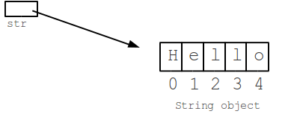# 4 Methods To Find Java String Length() | Str Length

Java program to calculate length of string – In this article, we will discuss the various methods to calculate the length of a string, str length in Java programming. String length() java has been written in 3 to 4 different ways, you can check out here. If you any queries about source code str length in java, leave a comment here.

• Using Standard Method
• Using Scanner Class (Predefined method)
• Using Scanner Class
• Using Static Method
• Using Separate Class

A string is a data type used in programming like an integer or a floating point but it is used to represent text whenever it is required instead of numerical.As you can see, the string mentioned here is “Hello”. The string consists of 5 characters. All of the 5 characters are represented by a pointer. The location is represented by a pointer, in this case, starting from 0 to 4.

Similarly, other strings also have ideal lengths where the space between two words is also counted.

## str length in Java – Using Standard Method

To find the length to string, we first taken in a string input in the code itself.

This string input is converted to a character array so as to traverse to the end of string individual character-wise to find the length.

To make this conversion, we can make use of a built in method for strings as below:

In this character array, we traverse until the end of the array in a loop and keep incrementing the count which is to be stored in a separate integer variable (a).

The end value of this variable (a) is nothing but, the length of our input string.

Output:

### Java String Length – Predefined Method

In the above example, we had given inputs manually and predetermined in the code itself and if any changes in value were to be made, the user has to go to the code and make changes.

To avoid this, Scanner class in Java can be made use of.

With the help of Scanner class any primitive type inputs can be read at runtime.

So, for our problem, we will read a string input. String in Java has many predefined methods in it. T

By making use of this predefined method and giving our input string as the String whose length is to be found, we can directly display the length in our output screen.

Output:

Using Scanner Class

In here, just like the explanation given above to make use of scanner class to read inputs at runtime, the same method can be used to read our input.

After getting the input, instead of using a predefined method, we can make use of the logic discussed in the beginning by converting the string to a character array.

Output:

#### Using Static Method

This technique is made use of for better readability. We have split up the code into two methods in the same class.

The main method is responsible for all the input and output operations. Input is taken in with the help of Scanner class.

After taking input, a separate static method (length) consisting of the set of statements for calculation of length of string is called.

This method (length) converts individual alphabet to character and increments the count to finally find the total length of the string.

After executing all the statements in it, returns the length of the string. The main logic is enclosed in a try block so that we can catch if any exception occurs like a null pointer, out of bound, etc.

Output:

x

## Java Program to Add Two Matrices – 4 Ways | Programs

Java program to add two matrices – The following Java Code will let you know ...